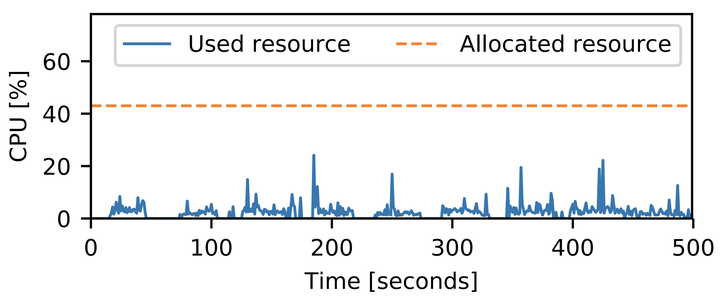# Improving Resource Utilization of Data Center using LSTMData centers are centralized facilities where computing and networking hardware are aggregated to handle large amounts of data and computation. In a data center, computing resources such as CPU and memory are usually managed by a resource manager. The resource manager accepts resource requests from users and allocates resources to their applications. A commonly known problem in resource management is that users often request more resources than their applications actually use. This leads to the degradation of overall resource utilization in a data center. This work aims to improve resource utilization in data centers by predicting the required resource for each application. We designed and implemented a neural network model based on Long Short-Term Memory (LSTM) to predict more efficient resource allocation for a job based on historical data.

A commonly known problem in resource management is over-allocation. Users often request more resources than their applications actually use. This degrades the overall resource utilization in data centers. The above figure compares the used and allocated resources in a Google’s data center. The plots clearly indicate that resources are wasted due to over-allocation.

## Methodology

The proposed model has two LSTM layers each of which learns the relationship between: (1) allocation and usage, and (2) CPU and memory. We used Googles cluster usage trace, which contains a trace of resource allocation and usage for each job executed on a Google data center, to train our neural network.

Design of our proposed prediction model based on LSTM. The model is composed of five main layers: (1) input layer, (2) first LSTM layer, (3) second LSTM layer, (4) fully connected layer and (5) output layer.

The first layer is the input layer which has a dimension of 4×1. The input layer receives the following four significant variables selected using PCA: (1) Requested CPU resource, (2) Requested memory resource, (3) Used CPU resource, and (4) Used memory resource. The second layer is the first LSTM layer that finds the correlation between the different computing resource types. This layer consists of two LSTM cells. The first cell focuses on CPU resource utilization, whereas the second cell focuses on memory resource utilization. The third layer is the second LSTM layer that finds the correlation between the different resource types. This layer also consists of two LSTM cells. The first cell focuses on allocated resources, whereas the second cell focuses on used resources.

We separate the two LSTM layers because the time-series information has to consider the value in the same space relation but the computing resource types and the resource utilization types are not in the same space relation. A multiplication layer is inserted between the first LSTM layer and the second LSTM layer to find the relationship between the computing resource space and the resource utilize space. The dimensions of both LSTM layers depend on the memory cell size because an LSTM layer requires its input size to be the same as the memory cell size.

The fourth layer is a fully connected layer to consider the significant features in each memorize time span which is based on the memory cell size. The dimension of a fully connected layer related to the memory cell size because it is layer after LSTM layer which has the dimension of output accord with the memory cell size. The last layer is the output layer that gives the suitable CPU allocation in the first cell and the suitable memory allocation.

## Evaluation

The resource manager supplies the resource request received from a user andthe resource usage in the data center to our prediction model.The prediction model then estimates the suitable resourceallocation. Based on this estimated resource allocation, theresource manager allocates computing resources.